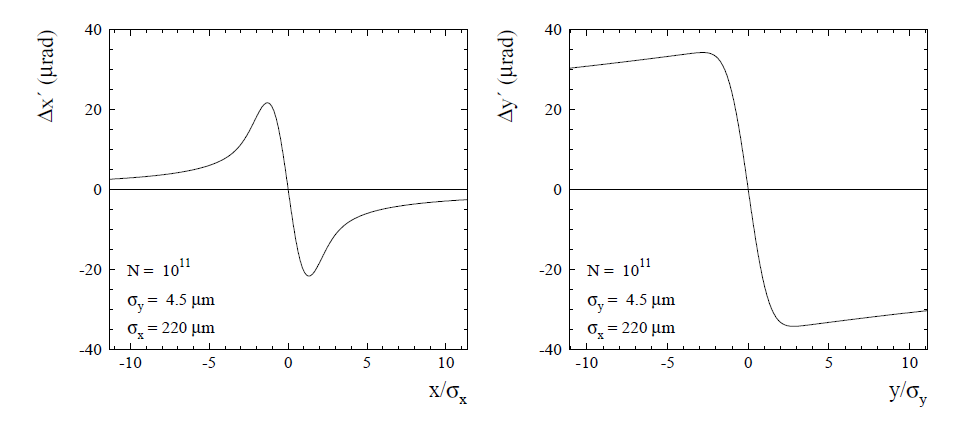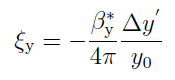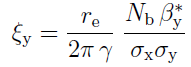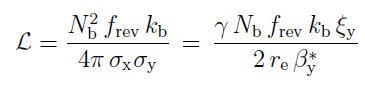# What characteristics are implied when you say that the machine operates at the beam-beam limit?

The beam-beam parameter (often also called beam-beam tune shift) characterizes the strength of the beam-beam force in a given plane on the particles of the opposing beam. The figues below show the deflection by the beam-beam force for the case of LEP (flat beams). Note the difference between x and y due to the fact that the horizontal beam size is much larger than the vertical size.The beam-beam parameter is measuring the linear part of the deflection (for small offsets wrt center of the bunch). For example for the vertical plane, the beam-beam parameter is given by:The beam-beam parameter can be expressed in terms of beam parameters. It depends on:

• the bunch population ~ N,
• the inverse of the energy ~ 1/gamma,
• the betatron function at the IP ~ beta*,
• the inverse of the beam size (sigma) products ~1/(sigmax*(sigmax+sigmay)) for horizontal plane x, ~1/(sigmay*(sigmax+sigmay)) for vertical plane y.

For flat beams (sigmax >> sigmay) the vertical beam-beam tune parameter can be approximated by:Because the beam-beam force is non-linear, it also introduces a spread of the tunes (--> beam-beam tune shift).

In a simple model: as long as the beam-beam force is below a critical value (the beam-beam limit), nothing happens. Above the limit the beam sizes start to blow up such a to maintain the beam-beam parameter equal to or smaller than the beam-beam limit value. Large tails develop, the lifetime goes down due to the tails. In that regime (at or above beam-beam limit) the luminosity no longer scales with N2, but only with N (due to the increase in beam size). Indeed one can express the luminosity as:When the beam-beam parameter is constant, the luminosity ~N !

In general one tries to operate the machine just below or at the beam-beam limit.

Category: